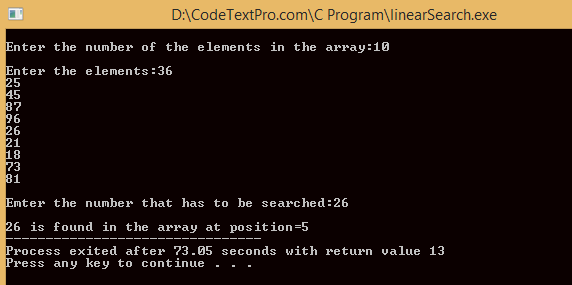# Linear Search Program in C || Algorithm of Linear SearchLinear Search Program/Algorithm

## What is searching?

### Linear Search:-

It is also known as sequential search. It works by comparing the value to be searched with every element of the array one by one in a sequence until a match is found.

### Linear Search Program in C

#include<stdio.h>

#include<conio.h>
main()

{

int arr, num, i, n, found=0, POS= -1;

printf("\n Enter the number of the elements in the array:");

scanf("%d", &n);

printf("\n Enter the elements:");

for(i=0; i<n; i++)
{
scanf("%d", &arr[i]);
}
printf("\n Emter the number that has to be searched:");

scanf("%d",&num);

for(i=0; i<n; i++)
{
if(arr[i]==num)
{
found=1;

POS=i;

printf("\n%d is found in the array at position=%d",num,i);

break;
}
}
if(found==0)

printf("\n%d does not exist in the array",num);

getch();

}

### Output of Program:Linear Search

### Algorithm of Linear Search

#### LINEAR_SEARCH(A, N, VAL)              --------->Function Name

Step1: [INITIALIZE] SET POS= -1

Step2: [INITIALIZE] SET I= 0

Step3: REPEAT STEP 4 WHILE I<=N

Step4: IF A[I] = VAL
SET POS = I
PRINT POS
GOTO STEP 6

[END OF IF]

SET I = I+1

[END OF LOOP]

Step5: IF POS = -1

PRINT " VALUE IS NOT PRESENT IN THE ARRAY "

[END OF IF]

Step6: EXIT